# paper on the unified theory

https://zenodo.org/record/4057270#.YQijLEApDIW

The preprint published on March 27, 2020

Unified Field Theory for Gravity and Electromagnetism

and Its Explanation for Dark Matter Observations

Max Gaofei Yan

wuji1116@gmail.com

Abstract

This paper defines the gravitational field as a medium with elastic properties, and the gravitational force occurs when matter creates a more concentrated region in the gravitational field. Further, this paper explains how electromagnetic waves are changing concentrations in this field. Hence, the gravitational field and electromagnetic field are the same, called the unified field. Based on this understanding of the unified field, there are attractive forces between electromagnetic waves as defined as:

F=Y1f1f2r2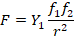There are also attractive forces between electromagnetic waves and matter possessing mass at rest. This force is defined as:

F=Y2fmr2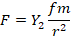Dark matter observations support this unified field theory.

Keywords: dark matter, gravitation, unified field theory, electromagnetic waves

Introduction

Since the Theory of Relativity by Albert Einstein was recognized, most people have accepted that the gravitational field can bend which can explain many natural phenomena. If the gravity field is defined as something that can stretch elastically, it can further explain the nature of gravity and many other related natural phenomena.

Section I – How Elastic Properties Create Attractive Forces

A flat perfectly elastic sheet will retain its original shape after subjecting it to stresses. While under no stress, the elastic sheet has no internal forces. While under stress, an elastic sheet’s internal forces act to retain the original shape of the sheet. This can be seen when a section of an elastic sheet is gathered and concentrated in a region while the entire sheet still stretches across the same area.

One method of creating such a concentrated region is with a knot. A knot made in the middle of the sheet will create outward pointing internal tension forces that surround the knot in all directions and act to undo the knot.Figure 1.1 – An elastic sheet with an evenly distributed concentration (left) and an elastic sheet with a knot in the center creating a concentrated region and outward pointing internal forces (right)

When there are two knots, both knots will create outward pointing internal tension forces that surround each in all directions. Because the tension forces point in all directions from each knot, there will be tension forces from each knot that point towards the other knot. These internal tension forces in the elastic sheet will create an attractive force between the two knots.Figure 1.2 – An elastic sheet with two knots and attractive internal forces between the two knots

This demonstrates the mechanism for how an attractive force is created between two knots in an elastic sheet.

Section II - Definition of Gravitational Fields and Gravitational Forces

The mechanism for the attractive force between two objects of matter in the gravitational field is similar to the mechanism described in Section I.

1. The gravitational field is defined as a medium that can stretch elastically to create a region of tension forces.

1. This elastic field will not always be even; it will have regions of concentrated and less concentrated, or thicker and thinner, gravitational field. Where matter is located, the field is more concentrated, or thicker. Where there is a void, the field is less concentrated, or thinner.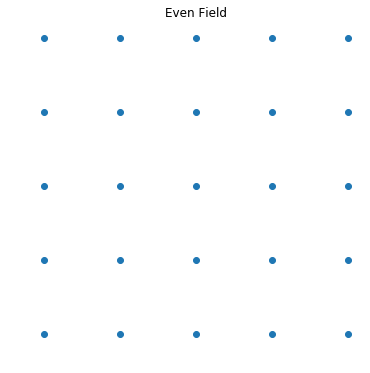Figure 2.1 – An even field with an evenly distributed concentration found in a void

1. Due to the elastic property of the gravitational field, a more concentrated region creates a tension surrounding it that pulls outward to even out the concentrated region.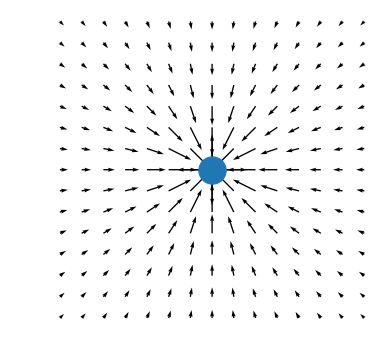Figure 2.2 – A dot representing matter that stretches the elastic field inward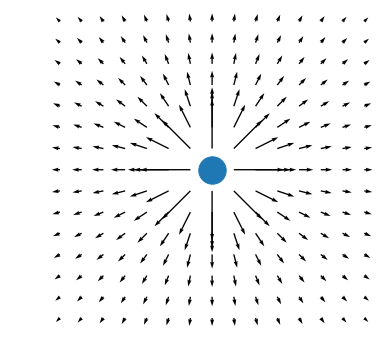Figure 2.3 – A dot representing matter and the force acting on the dot from the elastic field

Compared to the conventional idea of bending in 2D, the elastic field can be implemented in 3D in all directions as seen in Figures 2.4 and 2.5.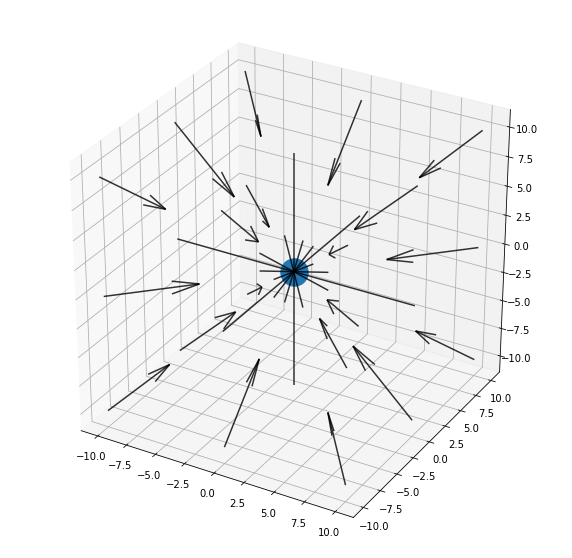Figure 2.4 – A dot representing matter that stretches the elastic field inward in a 3D space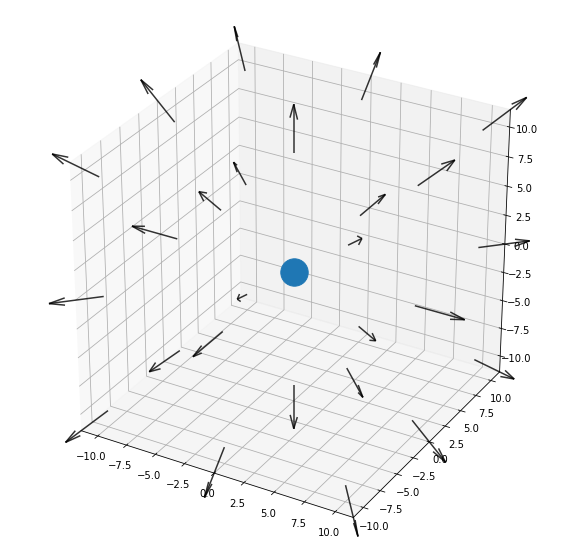Figure 2.5 – A dot representing matter and the force acting on the dot from the elastic field in a 3D space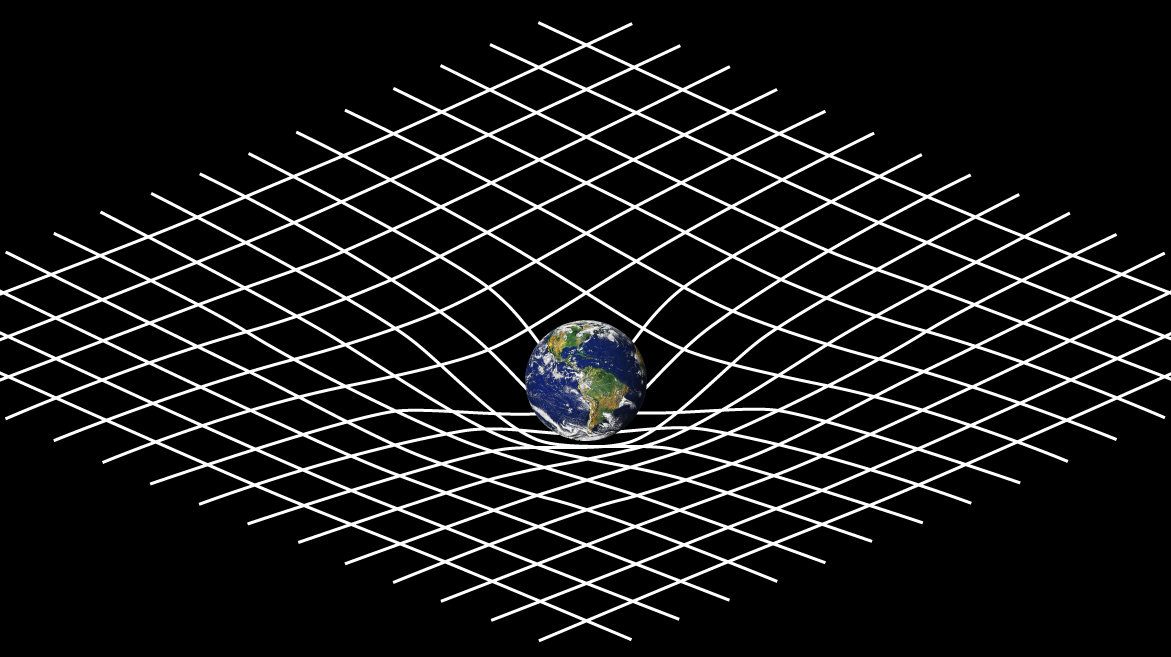Figure 2.6 – A traditional depiction of space time curvature from Einstein’s Theory of General Relativity      (NASA 2015)

The typical representation of Einstein’s General Relativity only shows a field in 2D, such as in Figure 2.6 above. If Einstein’s Theory of General Relativity were to be visualized in 3 dimensions, it would look like Figure 2.4. The idea of an elastic field can show stretching in all directions.

1. When there are two concentrated regions in the field, both create tension that pulls outward from each region as described in Point 3. From the surrounding tension forces created by each region, there will be tension forces from each region pull that towards each other in the elastic space. This attractive force between the two concentrated regions is the observed gravity force.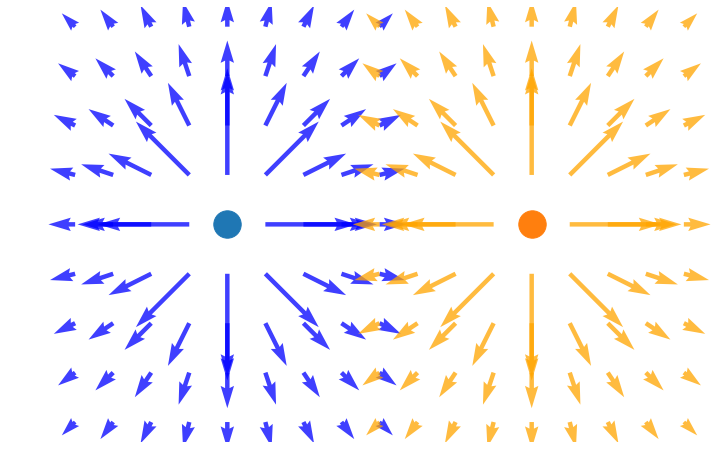Figure 2.7 – A region of tension forces from two objects of matter in a 2D space

When there is only one object of matter, there are forces around this object, but since the forces pull in all directions with the same magnitude, there are no observable effects. When a second object of matter enters the system, the effects of gravity can be observed as each objects’ gravitational field begins to pull on the other object in this elastic field, drawing the two objects closer.

1. Definition of the Gravity Force: The gravity force is the attractive force between two objects of matter due to tension between concentrated regions caused by both objects of matter in the elastic gravitational field.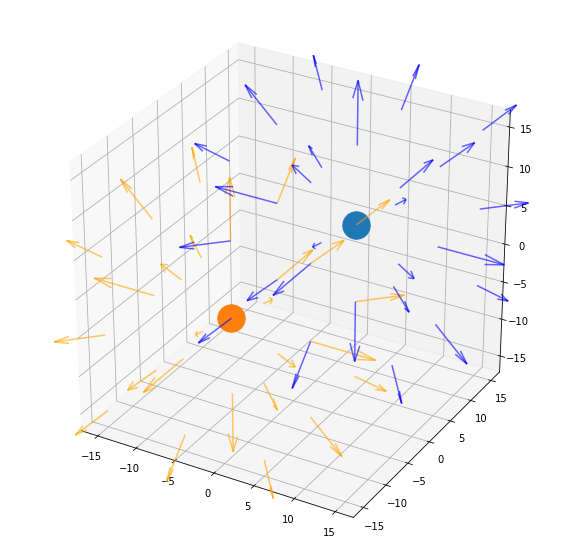Figure 2.8 – A region of tension forces from two objects of matter in a 3D space

Section III – Description of Electromagnetic Wave

1. Matter can be categorized as two categories: matter with mass at rest and matter with an invariant mass of zero. Electromagnetic waves fall into the latter category.

1. Electromagnetic waves are transverse waves in the elastic field described in Section II. At the same region, the transverse wave changes the concentration, or thickness, in the elastic field.

1. Electromagnetic waves propagate outward from a source in the elastic field much like ripples in water.

1. Electromagnetic waves are moving positions of changing concentrations in the elastic field described above in Section II.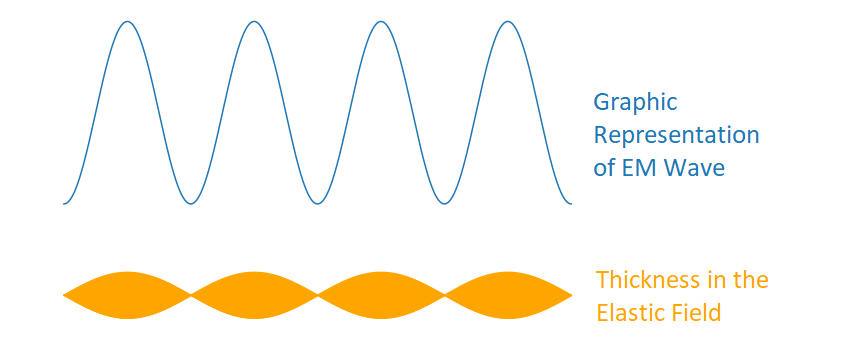Figure 3.1 – A 2D depiction of an electromagnetic wave and its corresponding thickness in the elastic field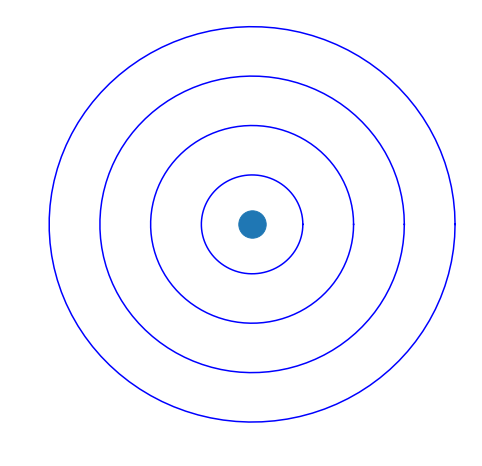Figure 3.2 – A 2D representation of a wave where the circles designate areas of higher concentration, or thickness, in the field

1. The electromagnetic field that allows for electromagnetic waves to travel is the same gravitational field described in Section II.

1. Because both gravitational forces and electromagnetic waves exist and operate using the elastic properties in the same field, this is a unified field for both gravity and electromagnetism.

Section IV – Gravitational Force Between Two EM Waves

1. Two EM waves have concentrated regions, according to the definition of gravitation in Section II, so they will have an attractive force acting between them, which is the gravitational force of two EM waves due to the elastic properties of the unified field.

1. By using Einstein’s Special Relativity, the matter with zero invariant mass can be calculated at the rest frame as its energy divided by the speed of light squared.

1. The force gravity between two photons at a certain point in time is as follows:

Given: The force of gravity, Einstein’s Mass–energy equivalence, and Planck’s Energy Frequency Relation

F=Gm1m2r2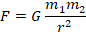E=mc2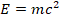or m=Ec2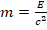E­=hf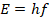F=Gm1m2r2=GE1c2E2c2r2= Ghf1hf2c4r2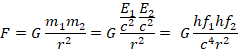(4.1)                                                           F= Gh2f1f2c4r2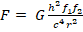Where:

F – is the force between two photons [kg m s-2]

G – is the gravitational constant [6.67e-11 m3 kg-1 s-2]

h – is Planck’s constant [6.63e-34 m2 kg s-1]

c – is the speed of light [3.00e8 m s-1]

r – is the distance between a photon in EM wave 1 and EM wave 2 [m]

f1 – is the frequency of a photon in EM wave 1 [s-1]

f2 – is the frequency of a photon in EM wave 2 [s-1]

A unit analysis of both sides of the equation shows that the units match.

F= mkgs2= m3kgs2×m2kgs2×1s×1s×ms-4×m-2=m7kg2s4m6kgs6=mkgs2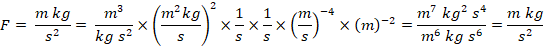Calculating the value of the constants, denoted as Y1, gives the following value:

(4.2)                          Y1=Fr2f1f2= Gh2c4=6.67×10-11m3kgs26.63×10-34m2kgs23.00×108ms4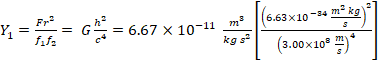(4.3)                                                   Y1=3.62×10-111m3kg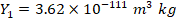This will give the equation:

(4.4)                                                               F=Y1f1f2r2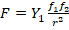A unitless multiple k can be included to denote the number of photons at a specific point in each of the two waves. This means that the photons will have to be the same distance away from the point in the other wave. This will give the final equation:

(4.5)                                                           F=Y1k1f1k2f2r2

Where:

F – is the force between two points of two EM waves [kg m s-2]

Y1 – is a constant [3.61e-111 m3 kg]

r – is the distance between a point in EM wave 1 and EM wave 2 [m]

f1 – is the frequency of a photon in EM wave 1 [s-1]

f2 – is the frequency of a photon in EM wave 2 [s-1]

k1 – is the number of photons at a point in EM wave 1

k2 – is the number of photons at a point in EM wave 2

Section V – Gravitational Forces Between Matter with Mass and EM Waves

1. According to the definition of gravitation in Section III, any two concentrated areas will create a gravitational force in the unified field, regardless if the concentration comes from matter that has mass at rest, like a planet, or matter with an invariant mass of zero, like an electromagnetic wave.

1. By using Einstein’s Special Relativity, the matter with zero invariant mass can be calculated at the rest frame as its energy divided by the speed of light squared.

1. The gravitational force between matter with mass at rest and matter an invariant mass of zero at a certain point in time is:

Given: The force of gravity, Einstein’s Mass–energy equivalence, and Planck’s Energy Frequency Relation

F=Gm1m2r2E=mc2or m=Ec2E­=hfF=Gm1m2r2=GEc2mr2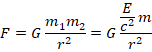(5.1)                                                             F=Ghfmc2r2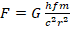Where:

F – is the force between a photon and an object with mass [kg m s-2]

G – is the gravitational constant [6.67e-11 m3 kg-1 s-2]

h – is Planck’s constant [6.63e-34 m2 kg s-1]

c – is the speed of light [3.00e8 m s-1]

r – is the distance between a photon and an object with mass [m]

f – is the frequency of a photon [s-1]

A unit analysis of both sides of the equation shows that the units match.

F= mkgs2= m3kgs2×m2kgs×1s×kg×ms-2×m-2=m5kg2s2m4kgs4=mkgs2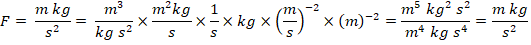Calculating the value of the constants, denoted as Y2, gives the following value:

(5.2)                               Y2=Fr2fm= Ghc2=6.67×10-11m3kgs26.63×10-34m2kgs3.00×108ms2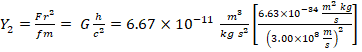(5.3)                                                 Y2=4.91×10-61m3s-1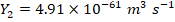This will give the equation:

(5.4)                                                             F=Y2fmr2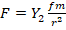A unitless multiple k can be included to denote the number of photons at a point in a wave. This means that the photons will have to be the same distance away from the object with mass. This will give the final equation:

(5.5)                                                           F=Y2kfmr2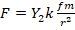Where:

F – is the force between a point in an EM wave and an object with mass [kg m s-2]

Y2 – is a constant [4.91e-61 m3 s-1]

r – is the distance between a point in the EM wave and an object with mass [m]

f – is the frequency of a photon [s-1]

m – is the mass of an object [kg]

k – is the number of photons at a point in the EM wave

1. Using the definitions of gravity forces and EM waves above, matter with mass will affect massless objects through this unified field. This is how celestial objects “bend” light. The body in space creates tension in this elastic field and pulls in the photons, altering their course.

1. The definition of gravity and EM waves leads to a very simple and direct explanation of why light bends around planets; it is because EM waves and gravitational fields operate using this elastic feature in the unified field.